### Repetition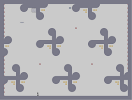Hover over the thumbnail for a full-size version.

Author MARIJNENANDRIES author:marijnenandries playable puzzle rated 2006-01-15 4 by 7 people. \$Repetition#MARIJNENANDRIES#none#00000000;10:=000001=00000000000:=0000000010000000000000000000;<010000000000000000000:11111<0;<000000000000000;10:=01=000000000000000:=00001000000000000000000000010000000000000000000000111<00000;<00000000000010:=000001=000000000000=0000000010000000000000000000;<010000000000000000000:11111<00000;<00000000000;10:=000001=00000000000:=0000000010000000000000000000;<010000000000000000000:11111<0;<000000000000000;10:=01=000000000000000:=00001000000000000000000000010000000000000000000000111<00000;<00000000000010:=000001=000000000000=0000000010000000000000000000;<010000000000000000000:11111<00000;<00000000000;10:=000001=00000000000:=0000000010000000000000000000;<010000000000000000000:11111<000000000000000000;10:=0|5^228,564!12^24,576!12^24,552!12^24,528!12^24,504!12^24,456!12^24,432!12^24,408!12^24,384!12^24,360!12^24,336!12^24,240!12^24,216!12^24,192!12^24,168!12^24,144!12^24,120!12^24,96!12^24,72!12^24,48!12^24,24!12^768,24!12^768,48!12^768,72!12^768,96!12^768,120!12^768,144!12^768,168!12^768,192!12^768,216!12^768,240!12^768,264!12^768,288!12^768,312!12^768,336!12^768,360!12^768,384!12^768,408!0^84,492!0^72,492!0^84,504!0^396,492!0^384,492!0^396,504!0^660,276!0^672,276!0^348,276!0^360,276!0^252,60!0^264,60!0^504,60!0^516,60!0^36,276!0^48,276!0^288,276!0^300,276!0^300,288!0^516,72!0^564,60!0^576,60!0^612,276!0^600,276!0^612,288!0^444,492!0^456,492!0^132,492!0^144,492!12^456,168!12^240,384!0^708,492!0^696,492!0^708,504!12^552,384!9^420,48,1,1,3,12,0,0,-1!11^204,60,420,48!7^132,144,3# A small jumper puzzle, all gold is pretty hard. Just try it. Challenge to any good puzzler :)

## Other maps by this author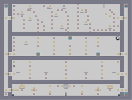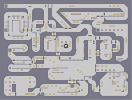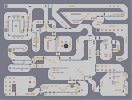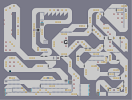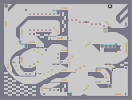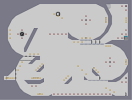Elevators Final Destination 2 Final destination improved Final Destination 3 WTF Flow Job